Chapter 15. Nuclear Chemistry

Learning Objectives

1. Express amounts of radioactivity in a variety of units.

Later in this chapter, in the section “Half-Life”, we use mass to indicate the amount of radioactive substance present. This is only one of several units used to express amounts of radiation. Some units describe the number of radioactive events occurring per unit time, while others express the amount of a person’s exposure to radiation.

Perhaps the direct way of reporting radioactivity is the number of radioactive decays per second. One decay per second is called one becquerel (Bq). Even in a small mass of radioactive material, however, there are thousands upon thousands of decays or disintegrations per second. The unit curie (Ci), now defined as 3.7 × 1010 decays/s, was originally defined as the number of decays per second in 1 g of radium. Many radioactive samples have activities that are on the order of microcuries (µCi) or more. Both the becquerel and the curie can be used in place of grams to describe quantities of radioactive material. As an example, the amount of americium in an average smoke detector has an activity of 0.9 µCi. (The curie is named after Polish scientist Marie Curie, who performed some of the initial investigations into radioactive phenomena in the early 1900s; the becquerel is named after Henri Becquerel, who discovered radioactivity in 1896.)

Example 15.1

A sample of radium has an activity of 16.0 mCi (millicuries). If the half-life of radium is 1,600 y, how long before the sample’s activity is 1.0 mCi?

Solution
The following table shows the activity of the radium sample over multiple half-lives:

Time in Years Activity
0 16.0 mCi
1,600 8.0 mCi
3,200 4.0 mCi
4,800 2.0 mCi
6,400 1.0 mCi

Over a period of 4 half-lives, the activity of the radium will be halved four times, at which point its activity will be 1.0 mCi. Thus it takes 4 half-lives, or 4 × 1,600 y = 6,400 y, for the activity to decrease to 1.0 mCi.

Test Yourself
A sample of radon has an activity of 60,000 Bq. If the half-life of radon is 15 h, how long before the sample’s activity is 3,750 Bq?

60 h

Example 15.2

A sample of radium has an activity of 16.0 mCi. If the half-life of radium is 1,600 y, how long before the sample’s activity is 5.6 mCi?

Solution
In this case, we do not have an exact number of half-lives, so we need to use the more complicated equation (seen later in this chapter, in the section “Half-Life”) and solve for time. If the initial amount is represented by 16.0 mCi and the final amount is 5.6 mCi, we have: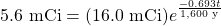To solve, we divide both sides of the equation by 16.0 mCi to cancel the millicurie units: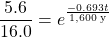By taking the natural logarithm of both sides; the natural logarithm cancels the exponential function. The natural logarithm of 5.6/16.0 is −1.050. So: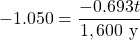The negative sign cancels, and we solve for t. Thus: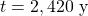It makes sense that the time is greater than one half-life (1,600 y) because we have less than one-half of the original activity left.

Test Yourself
A sample of radon has an activity of 60,000 Bq. If the half-life of radon is 15 h, how long before the sample’s activity is 10,000 Bq?

38.8 h

Other measures of radioactivity are based on the effects it has on living tissue. Radioactivity can transfer energy to tissues in two ways: through the kinetic energy of the particles hitting the tissue and through the electromagnetic energy of the gamma rays being absorbed by the tissue. Either way, the transferred energy — like the thermal energy from boiling water — can damage the tissue.

The rad (an acronym for radiation absorbed dose) is a unit equivalent to 1 g of tissue absorbing 0.01 J: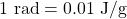Another unit of radiation absorption is the gray (Gy):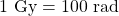The rad is more common. To get an idea of the amount of energy this represents, consider that the absorption of 1 rad by 70,000 g of water (approximately the same mass as a 150 lb person) would increase the temperature of the water by only 0.002°C. This may not seem like a lot, but it is enough energy to break about 1 × 1021 molecular C–C bonds in a person’s body. That amount of damage would not be desirable.

Predicting the effects of radiation is complicated by the fact that different types of emissions affect various tissues differently. To quantify these effects, the unit rem (an acronym for röntgen equivalent man) is defined as: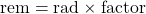where factor is a number greater than or equal to 1 that takes into account the type of radioactive emission and sometimes the type of tissue being exposed. For beta particles, the factor equals 1. For alpha particles striking most tissues, the factor is 10, but for eye tissue the factor is 30. Most radioactive emissions that people are exposed to are on the order of a few dozen millirems (mrem) or less; a medical X-ray is about 20 mrem. A sievert (Sv) is a related unit and is defined as 100 rem.

What is a person’s annual exposure to radioactivity and radiation? Table 15.1 “Average Annual Radiation Exposure (Approximate)” lists the sources and annual amounts of radiation exposure. It may surprise you to learn that fully 82% of the radioactivity and radiation exposure we receive is from natural sources — sources we cannot avoid. Fully 10% of the exposure comes from our own bodies — largely from carbon-14 and potassium-40.

Table 15.1 Average Annual Radiation Exposure (Approximate)
Source Amount (mrem)
medical sources 53
radioactive atoms in the body naturally 39
terrestrial sources 28
cosmic sources 28
consumer products 10
nuclear energy 0.05
Total 358

The actual effects of radioactivity and radiation exposure on a person’s health depend on the type of radioactivity, the length of exposure, and the tissues exposed. Table 15.2 “Effects of Short-Term Exposure to Radioactivity and Radiation” lists the potential threats to health at various amounts of exposure over short periods of time (hours or days).

Exposure (rem) Effect
1 (over a full year) no detectable effect
∼20 increased risk of some cancers
∼100 damage to bone marrow and other tissues; possible internal bleeding; decrease in white blood cell count
200–300 visible “burns” in skin, nausea, vomiting, fatigue
>300 loss of white blood cells; hair loss
∼600 death

One of the simplest ways of detecting radioactivity is by using a piece of photographic film embedded in a badge or a pen. On a regular basis, the film is developed and checked for exposure. Comparing the exposure level of the film with a set of standard exposures indicates the amount of radiation a person was exposed to.

Another means of detecting radioactivity is an electrical device called a Geiger counter (Figure 15.1 “Detecting Radioactivity”). It contains a gas-filled chamber with a thin membrane on one end that allows radiation emitted from radioactive nuclei to enter the chamber and knock electrons off atoms of gas (usually argon). The presence of electrons and positively charged ions causes a small current, which is detected by the Geiger counter and converted to a signal on a meter or, commonly, an audio circuit to produce an audible “click.”

Key Takeaways

• Radioactivity can be expressed in a variety of units, including rems, rads, and curies.

Exercises

# Questions

2. Define rem.
3. How does a becquerel differ from a curie?
4. Define curie.
5. A sample of radon gas has an activity of 140.0 mCi. If the half-life of radon is 1,500 y, how long before the activity of the sample is 8.75 mCi?
6. A sample of curium has an activity of 1,600 Bq. If the half-life of curium is 24.0 s, how long before its activity is 25.0 Bq?
7. If a radioactive sample has an activity of 65 µCi, how many disintegrations per second are occurring?
8. If a radioactive sample has an activity of 7.55 × 105 Bq, how many disintegrations per second are occurring?
9. A sample of fluorine-20 has an activity of 2.44 mCi. If its half-life is 11.0 s, what is its activity after 50.0 s?
10. Strontium-90 has a half-life of 28.1 y. If 66.7 Bq of pure strontium-90 were allowed to decay for 15.0 y, what would the activity of the remaining strontium-90 be?
11. How long does it take 100.0 mCi of fluorine-20 to decay to 10.0 mCi if its half-life is 11.0 s?
12. Technetium-99 is used in medicine as a source of radiation. A typical dose is 25 mCi. How long does it take for the activity to reduce to 0.100 mCi? The half-life of 99Tc is 210,000 y.
13. Describe how a radiation exposure in rems is determined.
14. Which contributes more to the rems of exposure — alpha or beta particles? Why?
15. Use Table 15.2 “Effects of Short-Term Exposure to Radioactivity and Radiation” to determine which sources of radiation exposure are inescapable and which can be avoided. What percentage of radiation is unavoidable?
16. Name two isotopes that contribute to the radioactivity in our bodies.
18. Explain how a Geiger counter works to detect radiation.

1. A unit of radioactive exposure equal to 0.01 J of energy per gram of tissue.
1. A becquerel is 1 decay/s, whereas a curie is 3.7 × 1010 decays/s.
1. 6.0 × 103 y
1. 2.41 × 106 disintegrations per second
1. 0.104 mCi
1. 36.5 s
1. By using a film badge, which is exposed by the radiation, or a Geiger counter.
1. Radioactive atoms in the body, most terrestrial sources, cosmic sources, and nuclear energy sources are likely unavoidable, which is about 27% of the total exposure. If exposure to radon gas is added, the total unavoidable exposure increases to 82%.
1. Film is exposed by the radiation. The more radiation film is subjected to, the more exposed it becomes.